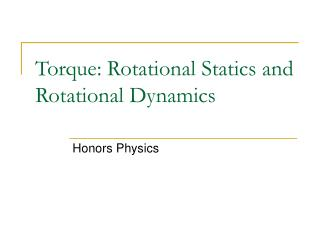DownloadDownload PresentationTorque: Rotational Statics and Rotational Dynamics

# Torque: Rotational Statics and Rotational Dynamics

Download Presentation## Torque: Rotational Statics and Rotational Dynamics

- - - - - - - - - - - - - - - - - - - - - - - - - - - E N D - - - - - - - - - - - - - - - - - - - - - - - - - - -
##### Presentation Transcript

1. Torque: Rotational Statics and Rotational Dynamics Honors Physics

2. Torque Torque is another rotational analog to Newton's’ laws, specifically Newton’s first and second law.

3. What is Torque? Torque is defined as the Force that is applied TANGENT to the circle and at some lever arm distance causing rotation around a specific point. Lever Arm Distance, r POR – Point of Rotation Circular Path of the handle

4. Torque TWO THINGS NEED TO BE UNDERSTOOD: 1) The displacement from a point of rotation is necessary. Can you unscrew a bolt without a wrench? Maybe but it isn't easy. That extra distance AWAY from the point of rotation gives you the extra leverage you need. THUS we call this distance the LEVER (EFFORT) ARM (r) . 2) The Force MUST be perpendicular to the displacement. Therefore, if the force is at an angle, sinq is needed to meet the perpendicular requirement.

5. Equation for Torque We use the Greek letter, TAU Anytime you have TWO VECTORS which MUST be perpendicular you have what is called a CROSS PRODUCT. The MAGNITUDE of the torque can be found using the equation above. The direction, however, requires some thought. • Counterclockwise rotation is considered to be POSITIVE which causes OUT OF THE PAGE torque. The direction of the torque is considered to be the direction that the BOLT moves. The bolt is being loosened. • Clockwise rotation is considered to be NEGATIVE which causes INTO THE PAGE torque. The bolt is being tightened down in this case.

6. Example A 150 kg man stands 2 m from the end of a diving board. How much torque does he apply at the bas where the board is attached assuming the board remains horizontal? Torque takes the units of Force and Displacement 2940 Nm

7. Torque and N.F.L. – “Rotational Equilibrium” According to Newton's first law, if an object is at rest it can be said to be in a state of static equilibrium. In other words, all of the FORCES cancel out to that the net force is equal to zero. Since torque is the rotational analog to force we can say that if a system is at rest, all of the TORQUES cancel out. r1 r2

8. Static Equilibrium Example r1 r2 Suppose a 4600 kg elephant were placed on a see-saw with a 0.025 kg mouse. The elephant is placed 10 meters from the point of rotation. How far from the point of rotation would the mouse need to be placed so that the system exists in a state of static equilibrium? 1.84 x 106 m or 1433 miles (certainly not practical)

9. Unbalanced Torques Find the net torque on the wheel in Figure about the axle through O, taking a = 8.00 cm and b = 25.0 cm. You might be tempted to use the 30 degrees in the figure. But the 12 N force is perpendicular to radius “a” and thus the angle between them is 90. 0.96 Nm 4.75 Nm cw 3.79 Nm Note: Writing the answer as -3.79 Nm implies that the direction is clockwise.

10. Example A hungry 700 N bear walks out on a beam in an attempt to retrieve some goodies hanging at the end. The beam weighs 200 N and is 6.00 m long; the goodies weigh 80 N (a) Draw a free body diagram, (b) When the bear is at 1.00 m. find the tension in the wire  POR These are forces at the hinge The weight of the beam itself is drawn at its center of mass

11. Example A hungry 700 N bear walks out on a beam in an attempt to retrieve some goodies hanging at the end. The beam weighs 200 N and is 6.00 m long; the goodies weigh 80 N (a) Draw a free body diagram, (b) When the bear is at 1.00 m. find the tension in the wire  342.56 N